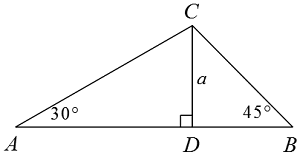# SAT Special Right Triangles

Geometry Level 1In the above figure, what is the length of $\overline{AB}$ in terms of $a?$

(A) $\ \ a\sqrt{3}$
(B) $\ \ 2a$
(C) $\ \ a + \sqrt{3}$
(D) $\ \ a + a\sqrt{2}$
(E) $\ \ a+a\sqrt{3}$

×